Published © GPL3+

# Golf Grip alignment tool

Having problems sinking your birdie putts? Maybe your putter grip is on crooked! This project gives you a way to perfectly align your grip.

BeginnerWork in progress5 hours1,744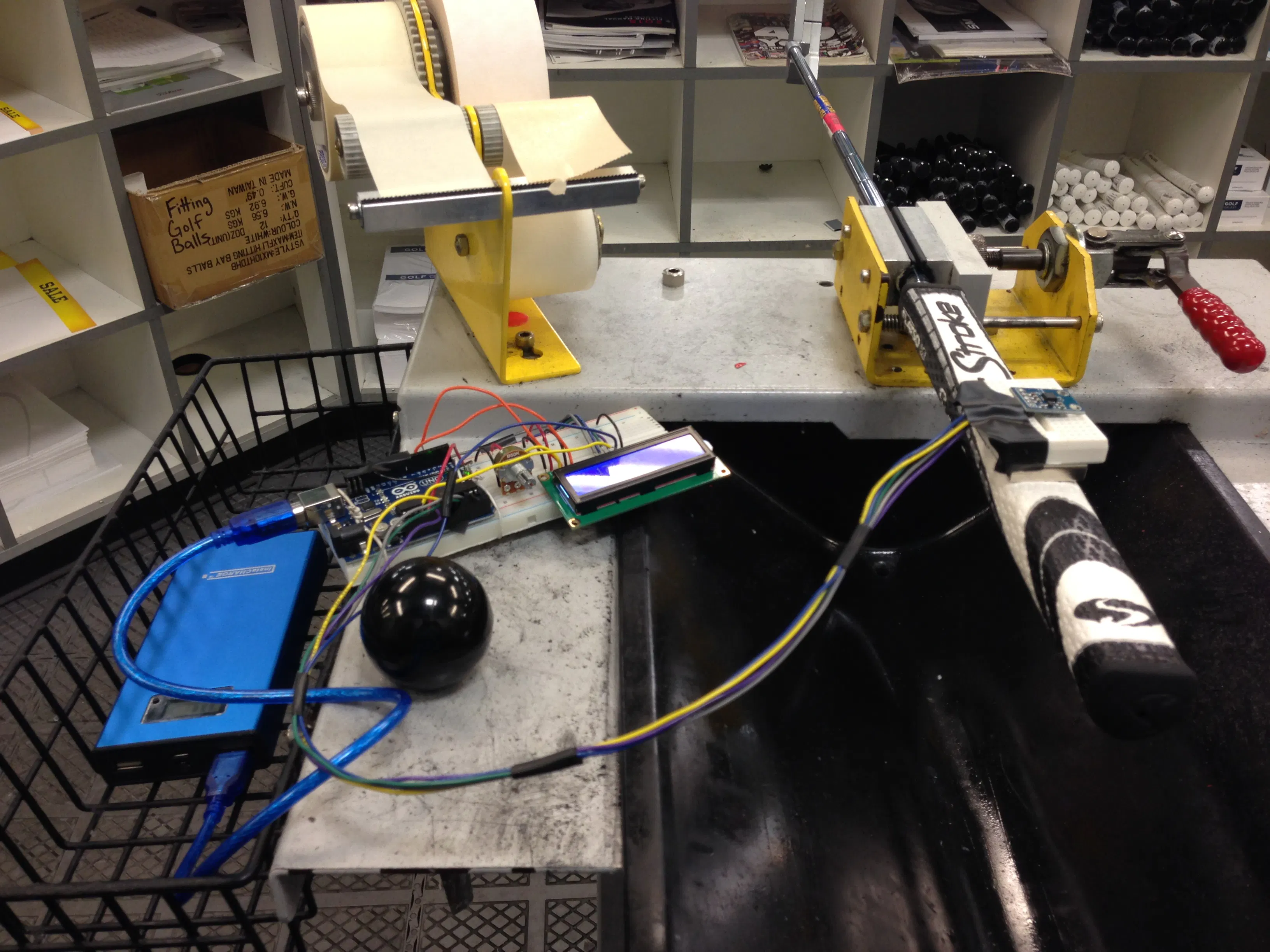## Things used in this project

### Hardware componentsBreadboard (generic)
×1Arduino UNO & Genuino UNO
×1Jumper wires (generic)
×1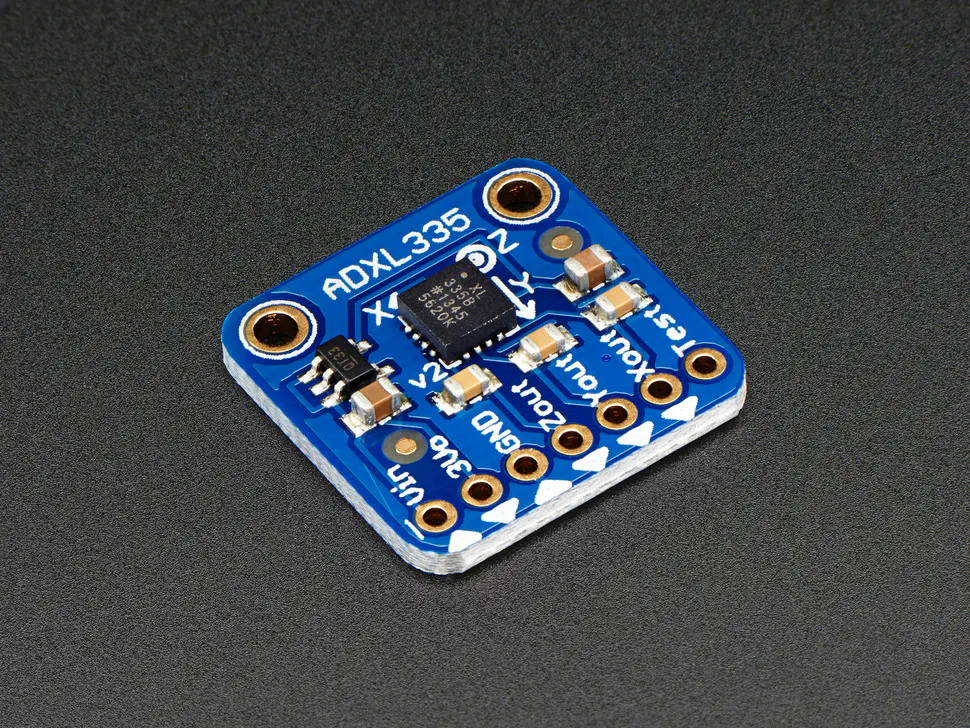Adafruit Analog Accelerometer: ADXL335
×1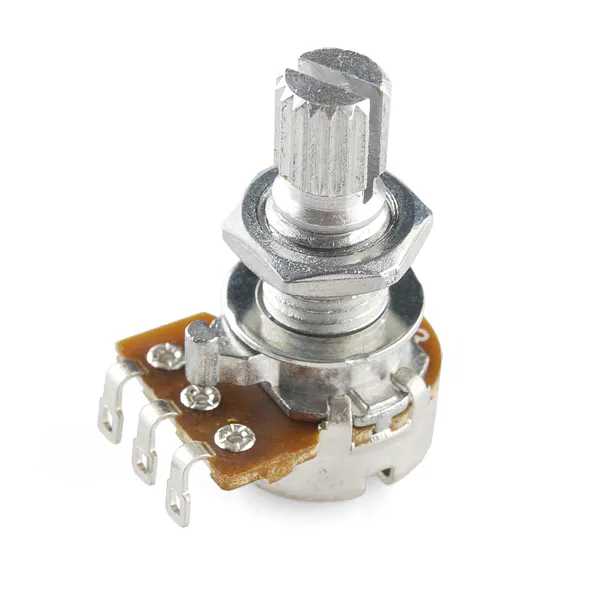Rotary potentiometer (generic)
×1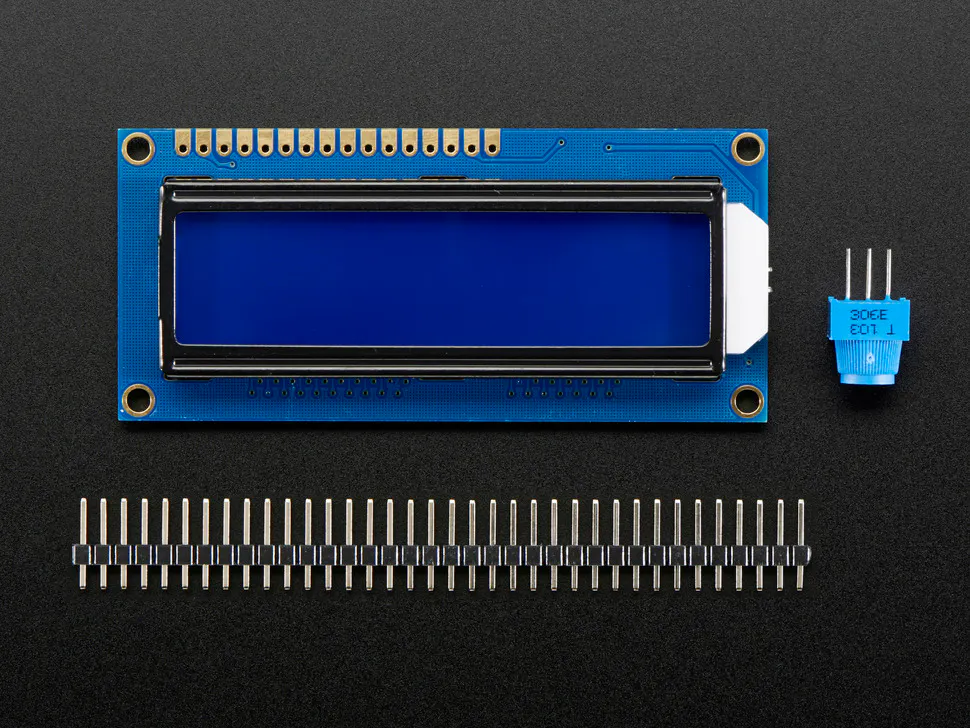Adafruit Standard LCD - 16x2 White on Blue
×1

### Software apps and online servicesArduino IDE

### Hand tools and fabrication machinesSoldering iron (generic)

Read more

## Schematics

### Circuit Schematic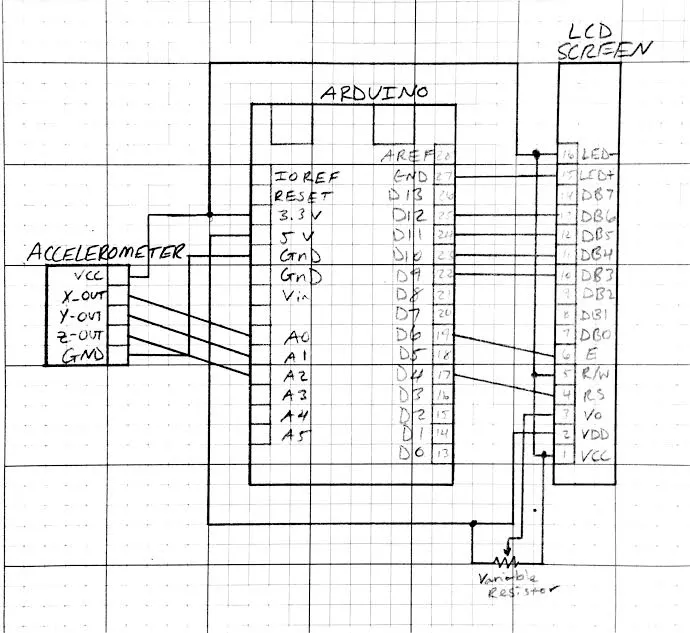### Installation Video

This is a video of a golf grip being installed. The accelerometer is used to make sure it is on straight!

## Code

### Accelerometer/lcd screen code

Arduino
This code reads the output from accelerometer and converts the output to degrees. It then sends the data to the LCD screen in the circuit and displays in degrees with respect to the X-axis of the acceleromter.
```#include <LiquidCrystal.h>// include the library code

/**********************************************************/
//char array1[]="  "; //the string to print on the LCD
//char array2[]="MONEY MAKER MIKE "; //the string to print on the LCD
int tim = 250; //the value of delay time
// initialize the library with the numbers of the interface pins
LiquidCrystal lcd(4, 6, 10, 11, 12, 13);

//Analog read pins
const int xPin = 0;
const int yPin = 1;
const int zPin = 2;

//The minimum and maximum values that came from
//the accelerometer while standing still
//You very well may need to change these
int minVal = 265;
int maxVal = 402;

//to hold the caculated values
double x;
double y;
double z;
double xa;
double xb;

void setup(){
Serial.begin(300);
lcd.begin(16, 2);
}

void loop(){

//read the analog values from the accelerometer
int xRead = analogRead(xPin);
int yRead = analogRead(yPin);
int zRead = analogRead(zPin);

//convert read values to degrees -90 to 90 - Needed for atan2
int xAng = map(xRead, minVal, maxVal, -90, 90);
int yAng = map(yRead, minVal, maxVal, -90, 90);
int zAng = map(zRead, minVal, maxVal, -90, 90);

//Caculate 360deg values like so: atan2(-yAng, -zAng)
//atan2 outputs the value of -π to π (radians)
//We are then converting the radians to degrees
x = RAD_TO_DEG * (atan2(-yAng, -zAng)+PI);
y = RAD_TO_DEG * (atan2(-xAng, -zAng) + PI);
z = RAD_TO_DEG * (atan2(-yAng, -xAng) + PI);

//Output the caculations
lcd.setCursor(0,0);
lcd.print("x: ");
lcd.print(xa);

xa = -180+abs(x);
xb = abs(xa);

if  (xa>178 || xb>178) //setting the tolerance

{
lcd.setCursor(0,2);
lcd.print("STOP!");
delay(600);
lcd.clear();
}

/*
lcd.print(" | y: ");
lcd.print(y);
lcd.print(" | z: ");
lcd.println(z);
*/

delay(300);//just here to slow down the serial output - Easier to read
}
```

## Credits

### Hunter Mitchell

1 project • 0 followers